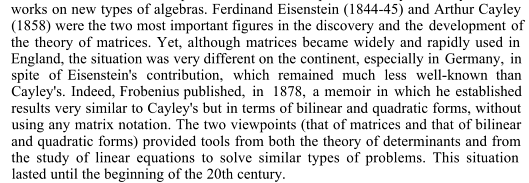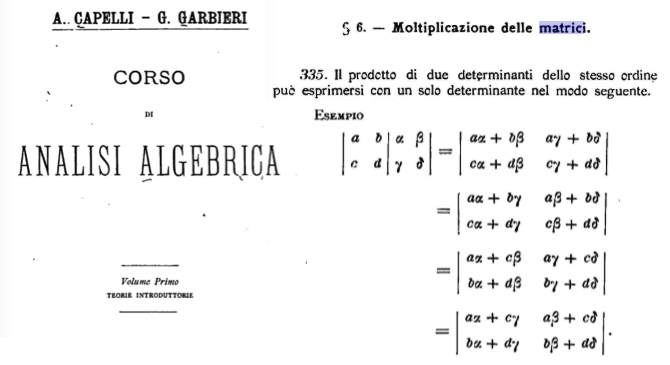# When exactly and why did matrix multiplication become a part of the undergraduate curriculum?

The story about Heisenberg inventing matrices and matrix multiplication in 1925 is very well known and well documented. A few weeks later, Born and Jordan read this work and recognized matrix multiplication, because one of them happened to take a course in "hypercomplex numbers" in his youth. This is how modern quantum mechanics was born.

This story clearly shows that in the second decade of the 20th century matrix multiplication was not something that every undergraduate was familiar with.

My questions:

1. When did this change? (Nowadays, I would say that this is the MOST standard part of the undergraduate curriculum in the US. More standard than Calculus. EVERY science or math major is taught to multiply matrices in her first year. It was similar in the Soviet Union, and I suppose this is the case everywhere). When did this dramatic change occur? When did linear algebra become a mandatory undergraduate subject?

But an even more interesting question is

1. WHY?

My conjecture is that this has something to do with the invention of Quantum mechanics. I have some arguments explaining this. But to test my arguments, I would like to know the answer to the first question, and other opinions on the second.

I know that matrix multiplication was introduced by Cayley (correct me if I am wrong), and that Hilbert-Courant was published in 1924. What physicists were using before Hilbert-Courant is not completely clear, but probably Thomson and Tait, or something similar. Of course, there is no mention of matrices in Thomson and Tait:-)

I understand that this may not be within the scope of this forum. Feel free to close. But I am asking in hope that someone by chance knows the answer to question 1, and will answer before the question is closed.

EDIT. Using Mathscinet, Zentralblatt and Jahrbuch, with "linear algebra", "lineare Algebra" etc. in the title, and year $$< 1950$$, I found a Turkish textbook of Pasha and Tefvik (1893), a textbook by H. Bohr and J. Mollerup (1938) and Russian text by Malcev (1949), from which I studied as an undergraduate. After 1950 there are many, which suggests that this curriculum revolution probably started in the early 1950s.

EDIT2: Google Ngram shows a sharp growth of the usage of the word "matrix", which begins in the middle 1940s. Against my expectation, it shows a peak in 1990 and then starts declining.

• @Per Alexandersson: To decide this we have to find an answer to the first question. Computer science is relatively young. – Alexandre Eremenko Nov 1 '14 at 23:45
• Do you really mean he invented matrices? After all, Cayley proved (what we now call) the Cayley-Hamilton theorem for size two and size three matrices in the 1850s, and a little later, determinants came into vogue; and I vaguely recall that Dodgson (Lewis Carroll) condemned the use of matrices in algebra. Perhaps you mean that he rediscovered matrices? – David Handelman Nov 2 '14 at 3:31
• Just to focus in, is your question about when US universities adopted linear algebra in their core curriculum? And, if this can be established, who were the advocates of this that made it happen? – David Hill Nov 2 '14 at 3:44
• Isn’t it a topic for matheducators.SE? – Incnis Mrsi Nov 2 '14 at 14:57
• Many here are suggesting that quantum mechanics played an important role, which is no doubt the case. However, the bigger part is most probably the rise of mathematical engineering, whose every facet is fundamentally driven by linear algebra. This includes everything from mechanical design, to every little part of electronic circuits, to structural finite elements, to linear and nonlinear controls, and so on so forth. – Richard Zhang Nov 3 '14 at 3:07

The article by J.-L. Dorier in On the Teaching of Linear Algebra suggests the answer to your question will be different for the UK and for continental Europe:In an attempt to answer your question more directly, I have searched for early University text books that introduce matrix multiplication. It was introduced in the context of the theory of determinants, to write the product of two determinants as a single determinant, in the text Corso di Analisi Algebrica from 1886. This is part 1 of the course, called "Introductory theories", so it may well have been intended for undergraduates.• The formula reported in your picture is not $C=AB$ but $C=A^TB$, and the Italian text translates to "The product of two determinants of the same order can be expressed as a single determinant as follows.". So someone with more free time than I have could argue that this is not a matrix product, but simply a composition rule for determinants that happens to be related to it. – Federico Poloni Nov 2 '14 at 17:41
• Well line 2 is the product. – Vincent Beffara Nov 2 '14 at 18:26
• @FedericoPoloni --- well, the title of this chapter is "Multiplication of Matrices" so this is definitely what the authors wanted to explain, but indeed, they do not differentiate between $AB$, $AB^T$, $A^TB$ and $A^TB^T$, because these matrix products all have the same determinant. So they do not insist that $AB$ is the "proper" way to multiply matrices, as we would do in a course today. – Carlo Beenakker Nov 2 '14 at 19:11
• Onetime I stmbled over a text "History of determinants" from the 1920's, which consistently talks about "the matrix of a determinant". – kjetil b halvorsen Dec 23 '17 at 10:54

Birkhoff and Mac Lane's 1941 "Survey of Modern Algebra" had a chapter on "The Algebra of Matrices". That book was influential in US curricula, and it would be a good place to look.

That answer is consistent with the influence of quantum mechanics, since quantum mechanics at Gottingen (as with Heisenberg) influenced algebra at Gottingen (as with Noether and van der Waerden), which in turn was the basis for Birkhoff and Mac Lane.

TL;DR: In the US, matrices and matrix multiplication probably became an expected part of the curriculum for engineering majors ca. 1970, and I would guess that the reason for this was its applications in electrical engineering and materials science.

19th Century: Through the 19th century, most physicists customarily wrote all equations component-wise. This covers Maxwell, and seems to have continued at least as late as 1905, because Einstein wrote his original paper on relativity in very awkward notation, without the use of vectors. There was a period when some theorists in physics were pushing a quaternionic system, but it was never widely used by physicists, much less by working electrical engineers. Hamilton's huge book on quaternions was known as the book that lots of people owned but nobody ever read.

Early 20th Century: The influential vector presentations of physics began when Gibbs and Heaviside simplified the useful aspects of the quaternionic system into the modern vector-scalar system, which was published in an influential 1901 text by Wilson. However, this seems to have taken a very long time to filter down into the curriculum. For instance, I have a copy of the 1927 edition of Elements of Physics, by Millikan and Gale. I believe this was the most popular introductory physics text in the US at that time. The word "vector" never appears, and we have on p. 64, "A force is completely described when its magnitude, its direction, and the point at which it is applied are given." In other words, the definition is such that forces don't even obey the ordinary law of vector addition, because there is a third datum, the point of application. There is no conception being presented of a more general abstract algebraic concept, just a specific definition of a force.

Middle 20th Century: I checked out some old UC Berkeley catalogs in order to see what the later historical situation was in the US.

In the 1959-60 catalog, there are two linear algebra courses in the math department:

• a lower-division course of linear algebra for social science majors
• an upper-division course that is part of a one-year algebra course for math majors

There were no linear algebra courses oriented at physical scientists or engineers. The engineering programs also did not offer a linear algebra class; they required mathematics courses only as prerequisites to the required engineering classes, and those prerequisites included vector calculus and differential equations, but no linear algebra.

In the 1969-70 catalog, there is a lower division linear algebra course, Math 11C. However, none of the required courses for an engineering degree seem to have 11C as a direct or indirect prerequisite. Probably by this time many engineering students were taking 11C -- otherwise I don't see why it would need to be moved from upper division to lower division -- but it wasn't required.

It's implausible that this change ca. 1970 had anything to do with Heisenberg's discovery ca. 1927 that quantum mechanics could be done using matrices. Not only is the gap in time very long, but if you look at modern treatments of quantum mechanics in physics books aimed at sophomore engineering majors, they don't make use of any linear algebra. In fact, it's more common to see matrices popping up when mutual inductance and mutual capacitance are introduced, but I think most texts do this without using the word "matrix" and without ever introducing matrix multiplication.

Current situation in the US: Here is my impression of the current situation in the US, which differs from the comments in the middle of question 1. At my school, the math sequence taken by STEM students goes like this: calculus of functions of a single variable; functions of several variables, including div, grad, and curl and differential equations; linear algebra. The linear algebra course is placed at the very end, presumably on the theory that it's a higher priority for engineering students to learn vector calculus in time for their course in electromagnetism.

Students in the US may get exposed to matrices in a high school pre-calculus course, but I don't think this is standard or highly emphasized. I believe that math textbooks used for functions of several variables usually assume no knowledge of linear algebra, even though this often makes the exposition of the subject extremely awkward.

• Regarding the "current situation in the US". At Georgia Tech (where I work) all engineers take 2 credit linear algebra usually as freshman or sophomores. All CS students take 4 credit linear algebra (and some CS threads also require a second course in linear algebra). We also have many engineering grad students taking review of linear algebra. – Igor Belegradek Jan 28 at 20:14
• @IgorBelegradek: Where is linear algebra placed in the sequence at your school? Is it after vector calculus? – Ben Crowell Jan 28 at 21:50
• Most Georgia Tech students transfer Differential Calculus credit, i.e., take it at high school. In the freshman year they usually take Integral Calculus and some flavor of linear algebra in no particular order. For many students linear algebra is their first math course at Georgia Tech. Linear algebra is a prerequisite for Multivariable Calculus and Differential equation, which engineers usually take (CS students do not). – Igor Belegradek Jan 28 at 22:06

There are some historical UK high school syllabuses at http://www.cambridgeassessment.org.uk/our-research/archives-service/past-exam-material/. Two by two matrices are in the 1984 O Level (taken at age 16) but not in the 1974 O Level or A Level (the latter taken at age 18). I don't know where you would find good data about undergraduate syllabuses.

• I think matrix multiplication was in year 3 (ie pre O-level) of the UK SMP Mathematics syllabus before 1967 (otherwise I was wasting my time learning it). – Geoff Robinson Jan 28 at 18:27